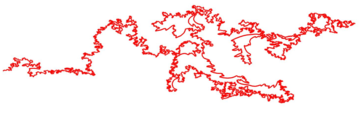# Core Courses## Advanced Topics in Stochastic Analysis

Dr Andreas Sojmark
(Imperial College)

This course will focus on three main areas:

1. Stochastic integration for processes with jumps
2. Weak and u.c.p. convergence theory for stochastic integrals
3. Interacting particle systems and McKean–Vlasov problems

## Advanced topics in Stochastic Processes

Professor Xue-Mei Li
(Imperial College)

This course will focus on Markov processes and related topics. We first review the basic concepts:

Feller Processes, Feller semigroups, Markov generators, Strong Markov processes, Strong Feller semi-groups and resolvents

We will then proceed to discuss topics chosen from the following list:

• Feynman-Kac formula and possibly higher order extensions
• Martingale problems
• Dynkin’s criterion and intertwining
• Convergence of stochastic processes
• Special classes of processes
• Connections with PDEs and Riemmanian structures
• Random dynamical systems
• Ergodic theorems

## Theories of Deep Learning

Prof Jared Tanner (Oxford)

Deep learning is the dominant method for machines to perform classification tasks at reliability rates exceeding that of humans, as well as outperforming world champions in games such as go.

Alongside the proliferating application of these techniques, the practitioners have developed a good understanding of the properties that make these deep nets effective, such as initial layers learning weights similar to those in dictionary learning, while deeper layers instantiate invariance to transforms such as dilation, rotation, and modest diffeomorphisms.

There are now a number of theories being developed to give a mathematical theory to accompany these observations; this course will explore these varying perspectives.

## Simulation Methods and Stochastic Algorithms

Prof. Mike Giles (Oxford)

This course will be an introduction to simulation methods for a wide range of stochastic models. The emphasis is on the construction of the numerical approximations with a limited discussion of their accuracy and computational cost, and almost no stochastic numerical analysis.

The topics covered include:

• random and quasi-random number generation;
• basics of Monte Carlo and Quasi-Monte Carlo simulation;
• Multilevel Monte Carlo and Multilevel QMC methods;
• sensitivity analysis;
• stochastic dierential equations;
• continuous-time Markov processes;
• stochastic PDEs;
• stochastic approximation;
• estimation of invariant measures;
• nested estimation;